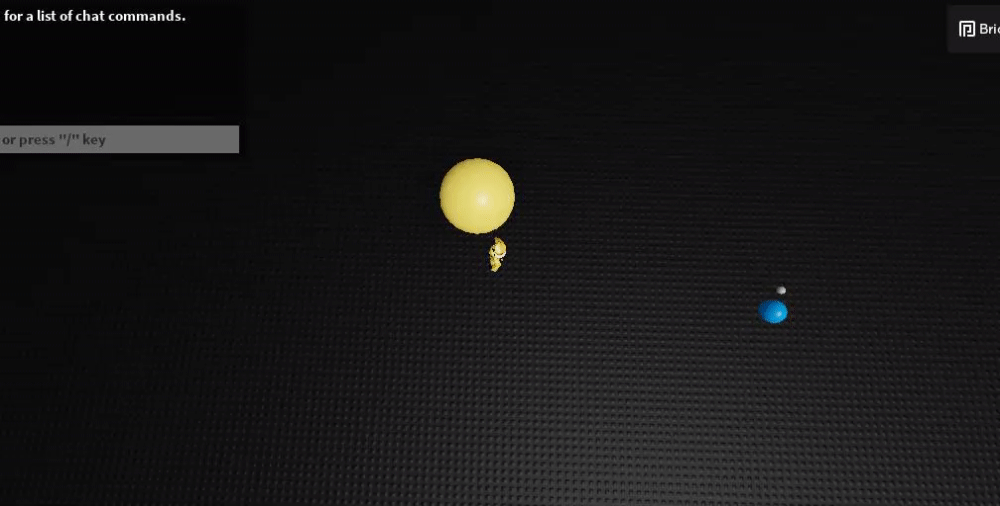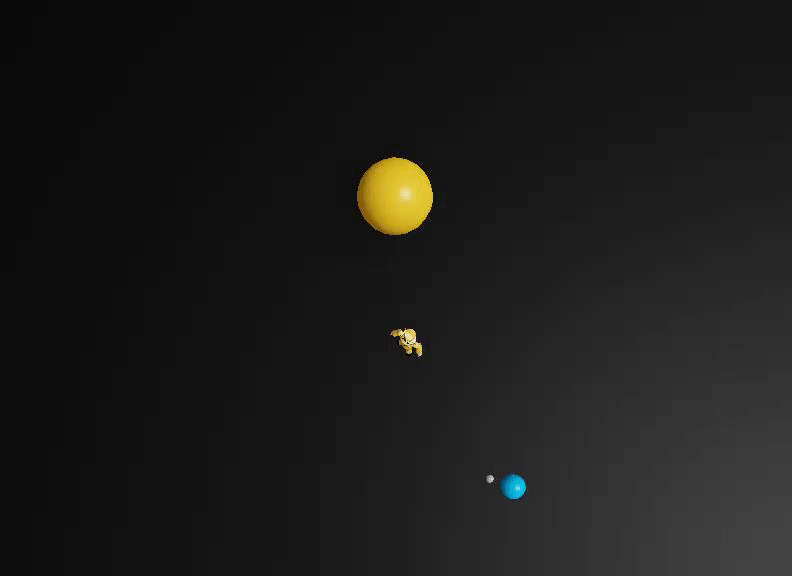# [Math] How can I rotate the earth around the sun while the moon rotating around the earth

I think there is not a big explanation. It will be like a orbit system

The Question: How can I rotate the earth around the sun while the moon rotating around the earth

IMPORTANT: PLEASE GIVE A EXPLANATION HOW YOU SOLVED UR CODE

For visualize things better:

1 Like

I think I can solve this with sin but I am not sure how to use it

Hi,
My approach to this problem was to calculate two different angles to get the position of each planetary body. Here’s the outcome:And here’s the code. I’ll take a moment to explain it so you can learn something from it. I’m positive this isn’t the only solution, nor is it necessarily accurate to how planets ACTUALLY move (I’m not a physicist), but I think this is what you were asking for:

``````local sun = workspace:WaitForChild("Sun")
local earth = workspace:WaitForChild("Earth")
local moon = workspace:WaitForChild("Moon")

local RunService = game:GetService("RunService")

local earthAngle, moonAngle = 0,0 --tracks the current rotation
local earthRotSpeed = 10 --arbitrary, degrees per second
local moonRotSpeed = 90 --arbitrary, degrees per second

local earthDistanceFromSun = 40
local moonDistanceFromEarth = 3

RunService.RenderStepped:Connect(function(dt)
if earthAngle >= 2*math.pi then
earthAngle -= 2*math.pi
end
if moonAngle >= 2*math.pi then
moonAngle -= 2*math.pi
end

earth.CFrame = sun.CFrame * CFrame.Angles(0, earthAngle, 0) * CFrame.new(earthDistanceFromSun, 0, 0)
moon.CFrame = earth.CFrame * CFrame.Angles(0, moonAngle, 0) * CFrame.new(moonDistanceFromEarth, 0, 0)
end)
``````

The code can be put anywhere, but it works best when in a LocalScript in StarterPlayerScripts (or anywhere on the client, really). So to briefly go over what I did here, you can see in the first two lines I simply defined sun, earth, and moon to be physical parts in the workspace.

Next, I define RunService which is a built-in service in Roblox that’ll help us with timing in this case.

The next three lines define the angles, and the rotation speed of the earth and moon. Those numbers are completely made up and can be whatever you want them to be. They represent degrees per second. earthAngle and moonAngle represent the initial rotation but it’s fine to keep them both zero.
After that on lines 11 and 12, we define the distance we want the earth to be from the sun, and the distance we want the moon to be from earth. Note that the center of each celestial body is how the distance is calculated, so if you leave the distance as 0 they will be occupying the same exact location.

The big part of this code is the call to the RunService.RenderStepped event, which runs every ‘step’, which for the purposes of this code is just a unit of time. We can get how long that ‘step’ took (the delta time, or dt as we name it), and add it to our earth and moon angles times the rotation speed to get a new angle for our current frame in time. The if statements are just there to clamp the rotation so that it never gets bigger than 2pi, which works because 2pi and 0 are the same angles on a circle (a full rotation). Lastly, we use CFrames to position the planet and moon by using a starting point (the sun for the earth, the earth for the moon). We multiply that starting CFrame by our rotation (in the Y-axis only) and then translate it by the distances we set on lines 11 and 12 in the X-axis.

That’s probably a lot to take in, I suggest taking some time to draw out a diagram of the situation with math to get a clearer picture of what’s going on.

To address your comment about solving this using sin, you totally can! You can write a set of parametric equations to get a circle:
`x = rcos(t)` and `y = rsin(t)`
although I didn’t go over it in my code, you can totally use those equations to solve this problem in a different way! Feel free to look up “Parametric Equations of a Circle”

9 Likes

Hey,

Roblox has a method for this specific gravity (Planetary Gravity). Line forces are really efficient and are more expected to take less memory

Example of Line Forces:

X = Sun Y = Planet

Y is attracted to X

Here is a video explaining it: Simulating Planetary Gravity using Line Forces! - Roblox Studio - YouTube

This would be great if you want to take a non-scripting approach!

Thanks! I totally understood it, but you did set it to -= 2*math.pi . Whats the sense behind hit could you demonstrate me that visual?

ahhhh because the rotation starts new?

So you did make full circle “…>= 2* math.pi…” and after that you did a newstart with -= 2*math.pi.

``````if earthAngle >= 2*math.pi then
earthAngle -= 2*math.pi
end
if moonAngle >= 2*math.pi then
moonAngle -= 2*math.pi
end
``````

So how would I do that with, x = rcos(t) and y = rsin(t)? Sorry but I never worked with rcos and rsin in Roblox Studio. I have a plan how I could do that but nomore. To afraid of getting brain stress xD - (hope you take it as humor).

Sorry for the later response but yes that was the purpose of that segment, otherwise the numbers will just keep getting bigger which isn’t ideal in code. Nice to keep the range between 0 - 2pi.

So with the parametric approach, you would first identify what all of the variables mean. x of course is the x-coordinate in this case, and y is the y-coordinate (this is for 2D, which works in this situation).
r is the radius, which would be similar to my original code using the `earthDistanceFromSun` and `moonDistanceFromEarth` variables (those are technically the r values you’d use)

All that is left, then, is t. That could simply be a time variable. An implementation of parametric equations might look something like this:

``````local sun = workspace:WaitForChild("Sun")
local earth = workspace:WaitForChild("Earth")
local moon = workspace:WaitForChild("Moon")

local RunService = game:GetService("RunService")

local earthRotSpeed = 10 --arbitrary, degrees per second
local moonRotSpeed = 90 --arbitrary, degrees per second

local earthDistanceFromSun = 40
local moonDistanceFromEarth = 3

local t = 0 -- time

RunService.RenderStepped:Connect(function(dt)
t += dt
local xEarth = sun.Position.X + earthDistanceFromSun * math.cos(t*math.rad(earthRotSpeed))
local zEarth = sun.Position.Z + earthDistanceFromSun * math.sin(t*math.rad(earthRotSpeed))

earth.CFrame = CFrame.new(xEarth, sun.Position.Y, zEarth)

local xMoon = earth.Position.X + moonDistanceFromEarth * math.cos(t*math.rad(moonRotSpeed))
local zMoon = earth.Position.Z + moonDistanceFromEarth * math.sin(t*math.rad(moonRotSpeed))

moon.CFrame = CFrame.new(xMoon, sun.Position.Y, zMoon)
end)
``````

You can see for each planetary body, the x and z (y if you were on a 2D graph, but z in this case since we are working with a horizontal plane in 3D) coordinates are defined based on the sun’s initial position (or earth for the moon) which acts as the origin, and then added to that are essentially the r * cos(t) and r * sin(t), but just using different variable names of course. You can then set the CFrame of each body with the x, y (sun’s y coordinate), and z values you get for each one.
It gives basically the exact same result:3 Likes